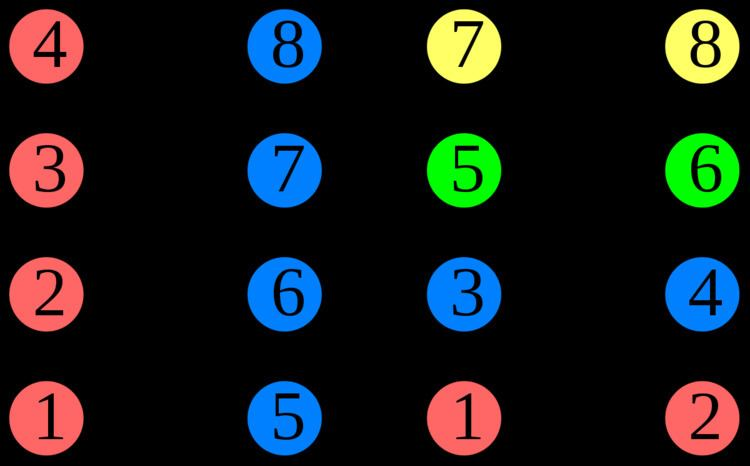Greedy coloring

Updated on
Covid-19In the study of graph coloring problems in mathematics and computer science, a greedy coloring is a coloring of the vertices of a graph formed by a greedy algorithm that considers the vertices of the graph in sequence and assigns each vertex its first available color. Greedy colorings do not in general use the minimum number of colors possible. However, they have been used in mathematics as a technique for proving other results about colorings and in computer science as a heuristic to find colorings with few colors.

Greed is not always good

A crown graph (a complete bipartite graph Kn,n, with the edges of a perfect matching removed) is a particularly bad case for greedy coloring: if the vertex ordering places two vertices consecutively whenever they belong to one of the pairs of the removed matching, then a greedy coloring will use n colors, while the optimal number of colors for this graph is two. There also exist graphs such that with high probability a randomly chosen vertex ordering leads to a number of colors much larger than the minimum. Therefore, it is of some importance in greedy coloring to choose the vertex ordering carefully. The number of colors produced by the greedy coloring for the worst ordering of a given graph is called its Grundy number.

It is NP-complete to determine, for a given graph G and number k, whether there exists an ordering of the vertices of G that forces the greedy algorithm to use k or more colors. In particular, this means that it is difficult to find the worst ordering for G.

Optimal ordering

The vertices of any graph may always be ordered in such a way that the greedy algorithm produces an optimal coloring. For, given any optimal coloring in which the smallest color set is maximal, the second color set is maximal with respect to the first color set, etc., one may order the vertices by their colors. Then when one uses a greedy algorithm with this order, the resulting coloring is automatically optimal. More strongly, perfectly orderable graphs (which include chordal graphs, comparability graphs, and distance-hereditary graphs) have an ordering that is optimal both for the graph itself and for all of its induced subgraphs. However, finding an optimal ordering for an arbitrary graph is NP-hard (because it could be used to solve the NP-complete graph coloring problem), and recognizing perfectly orderable graphs is also NP-complete. For this reason, heuristics have been used that attempt to reduce the number of colors while not guaranteeing an optimal number of colors.

Heuristic ordering strategies

A commonly used ordering for greedy coloring is to choose a vertex v of minimum degree, order the remaining vertices, and then place v last in the ordering. If every subgraph of a graph G contains a vertex of degree at most d, then the greedy coloring for this ordering will use at most d + 1 colors. The smallest such d is commonly known as the degeneracy of the graph.

For a graph of maximum degree Δ, any greedy coloring will use at most Δ + 1 colors. Brooks' theorem states that with two exceptions (cliques and odd cycles) at most Δ colors are needed. One proof of Brooks' theorem involves finding a vertex ordering in which the first two vertices are adjacent to the final vertex but not adjacent to each other, and each subsequent vertex has at least one earlier neighbor. For an ordering with this property, the greedy coloring algorithm uses at most Δ colors.

Alternative color selection schemes

It is possible to define a greedy coloring algorithm in which the vertices of the given graph are colored in a given sequence but in which the color chosen for each vertex is not necessarily the first available color; alternative color selection strategies have been studied within the framework of online algorithms. In the online graph-coloring problem, vertices of a graph are presented one at a time in an arbitrary order to a coloring algorithm; the algorithm must choose a color for each vertex, based only on the colors of and adjacencies among already-processed vertices. In this context, one measures the quality of a color selection strategy by its competitive ratio, the ratio between the number of colors it uses and the optimal number of colors for the given graph.

If no additional restrictions on the graph are given, the optimal competitive ratio is only slightly sublinear. However, for interval graphs, a constant competitive ratio is possible, while for bipartite graphs and sparse graphs a logarithmic ratio can be achieved. Indeed, for sparse graphs, the standard greedy coloring strategy of choosing the first available color achieves this competitive ratio, and it is possible to prove a matching lower bound on the competitive ratio of any online coloring algorithm.

Greedy coloring Wikipedia

Topics